# NCERT Solutions for Class 9 Maths Chapter 15 Exercise 15.1 – Probability

Download NCERT Solutions for Class 9 Maths Chapter 15 Exercise 15.1 – Probability. This Exercise contains 13 questions, for which detailed answers have been provided in this note. In case you are looking at studying the remaining Exercise for Class 9 for Maths NCERT solutions for other Chapters, you can click the link at the end of this Note.

### NCERT Solutions for Class 9 Maths Chapter 15 Exercise 15.1 – Probability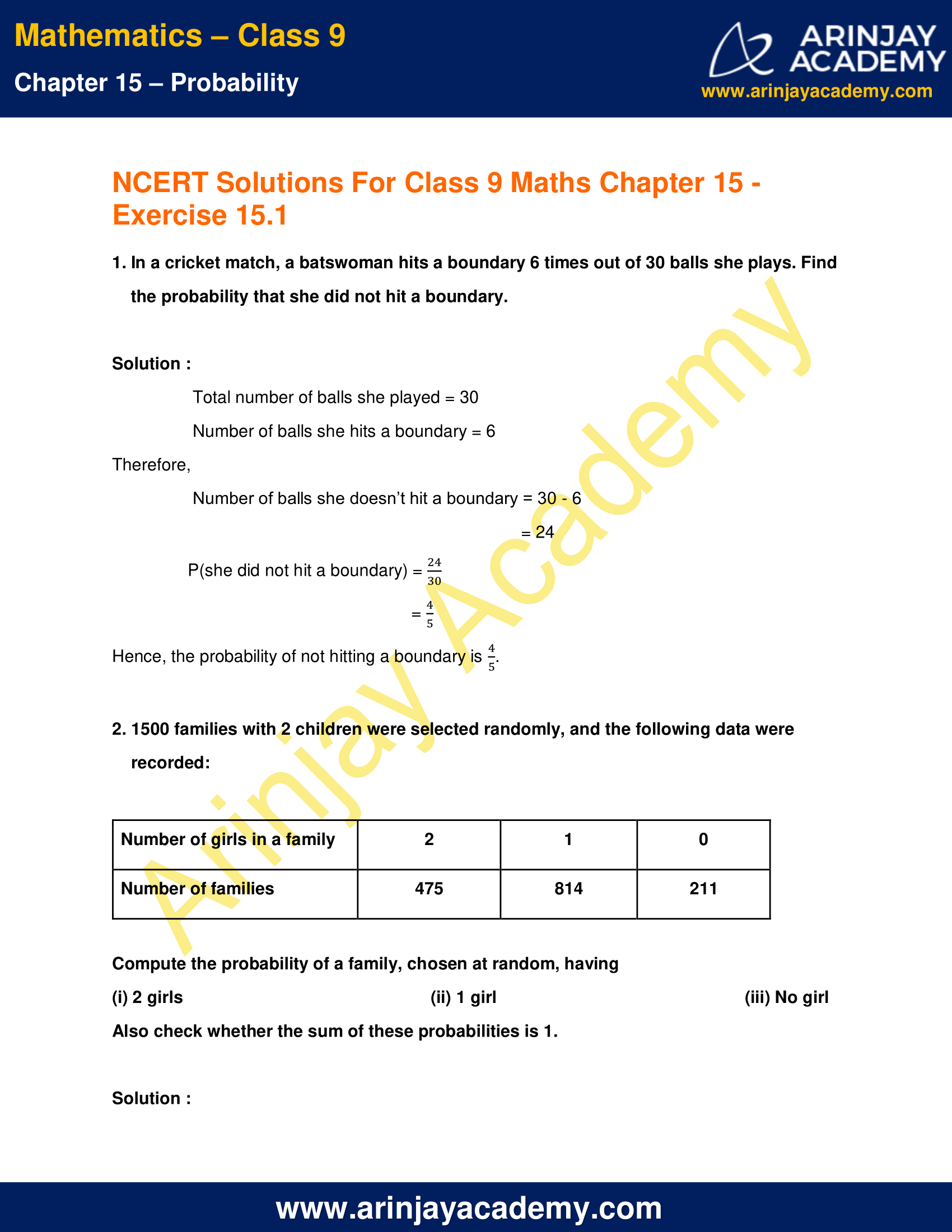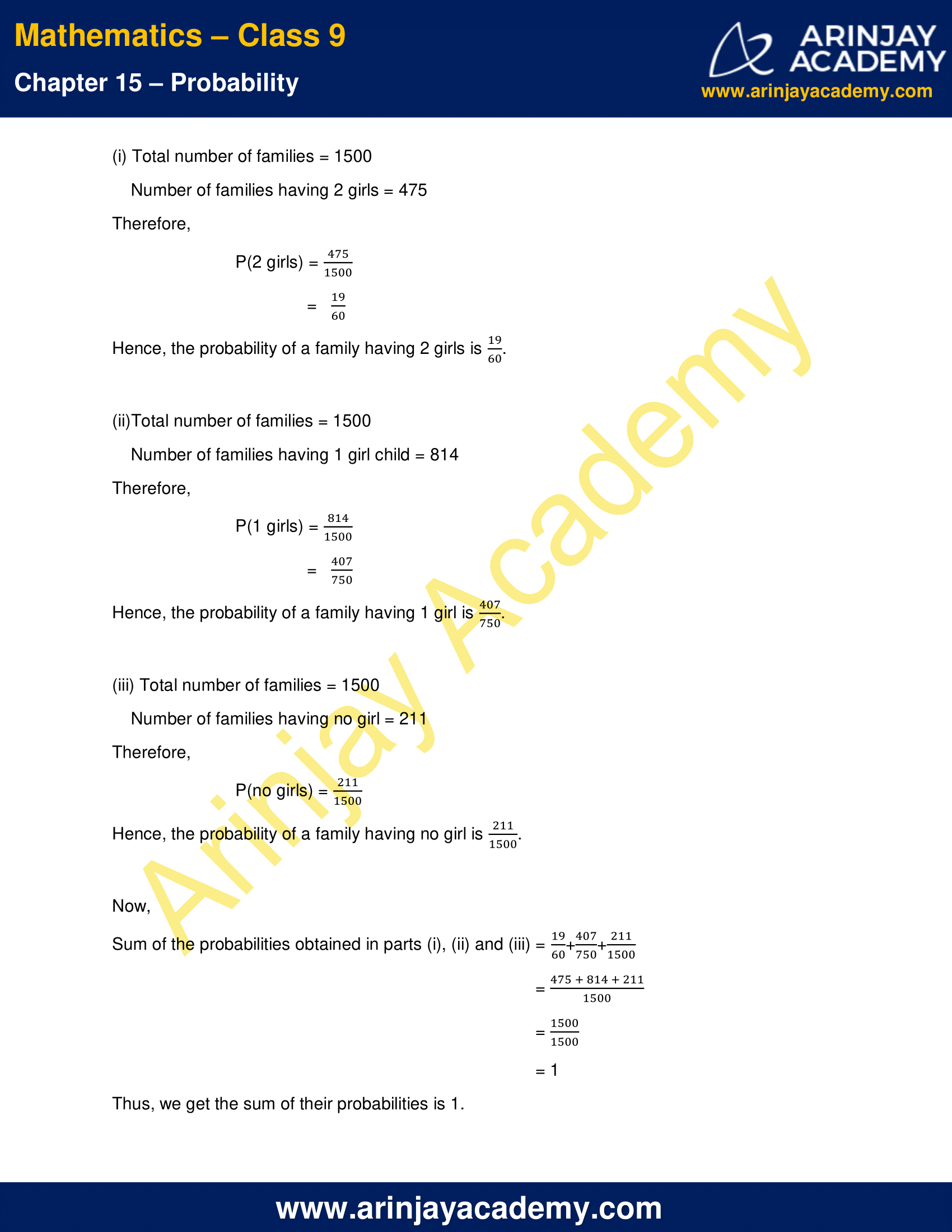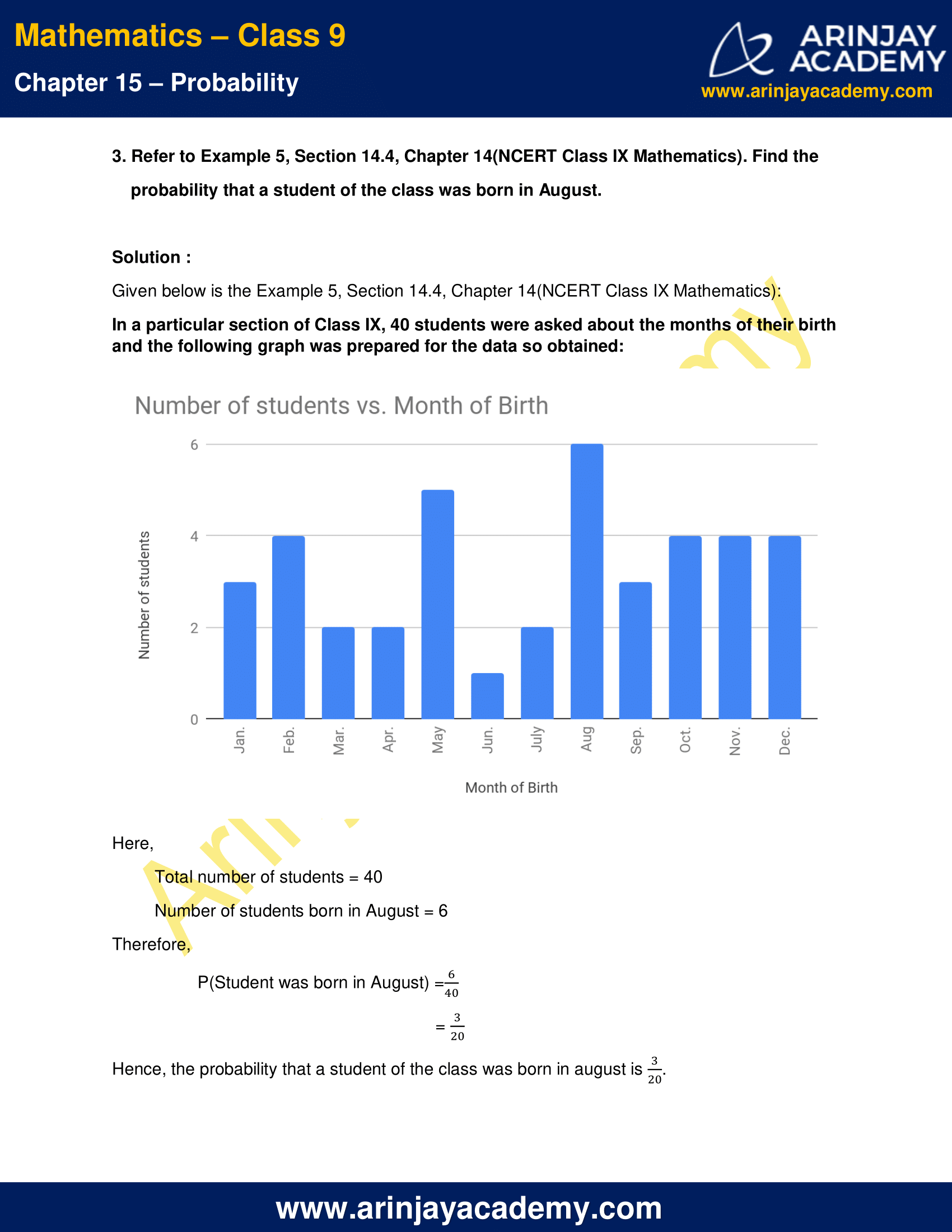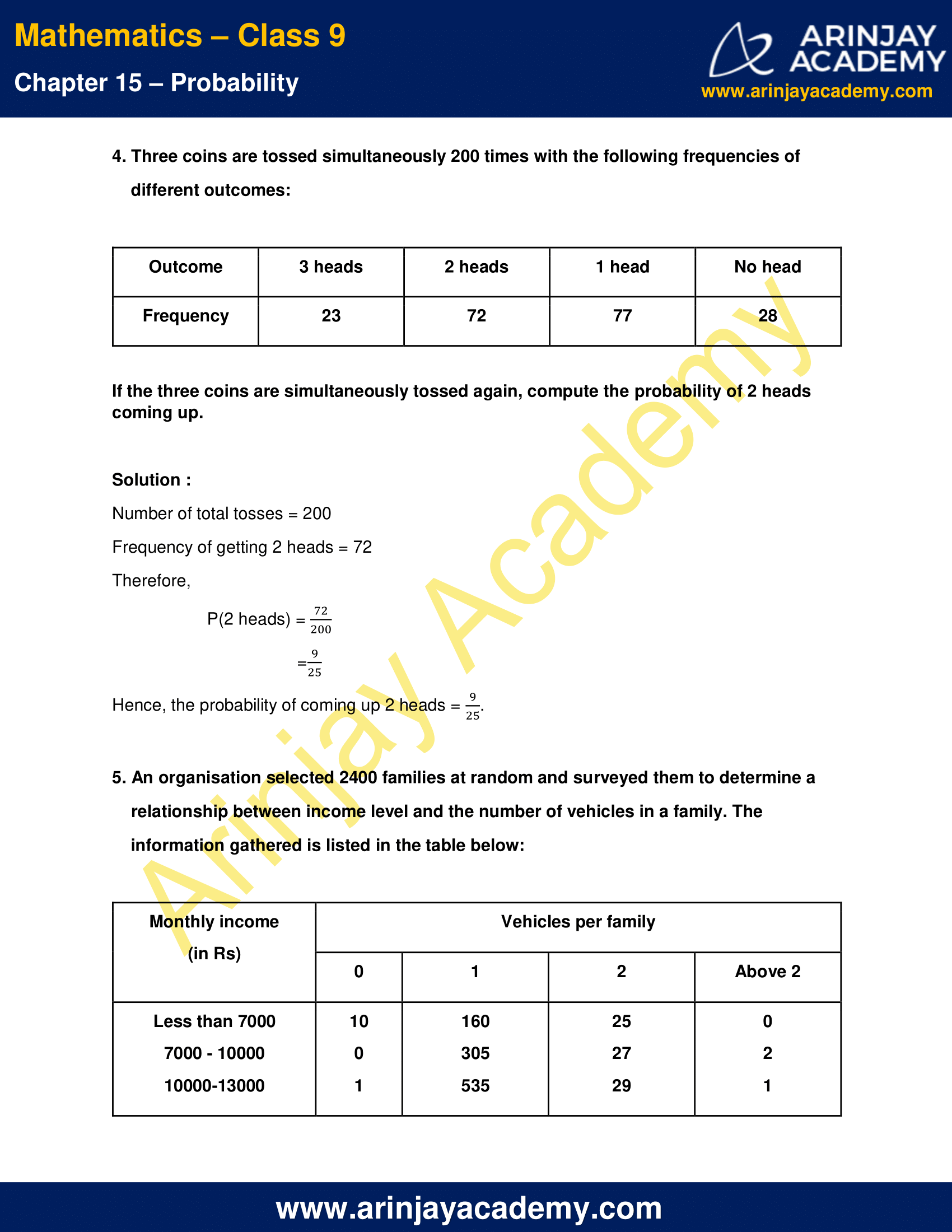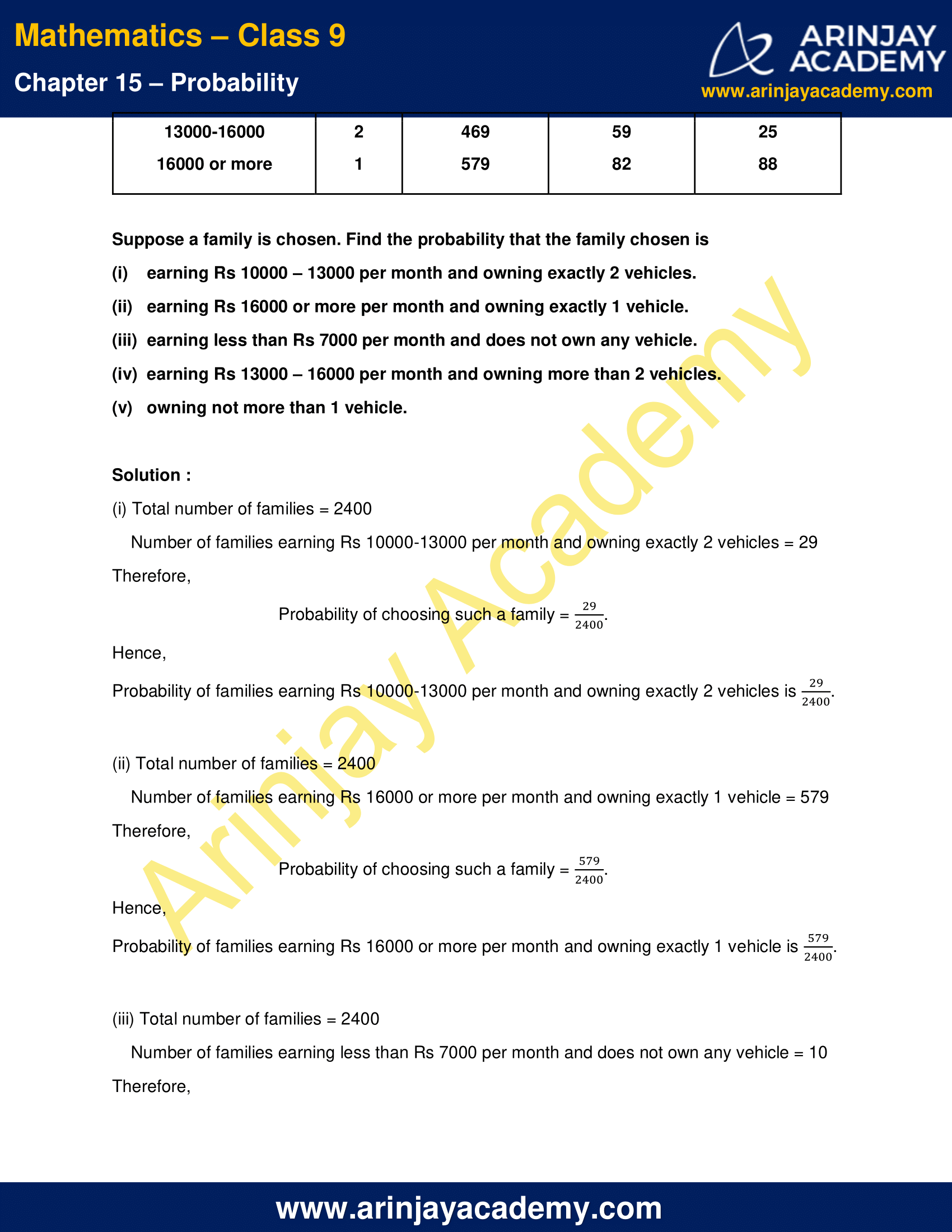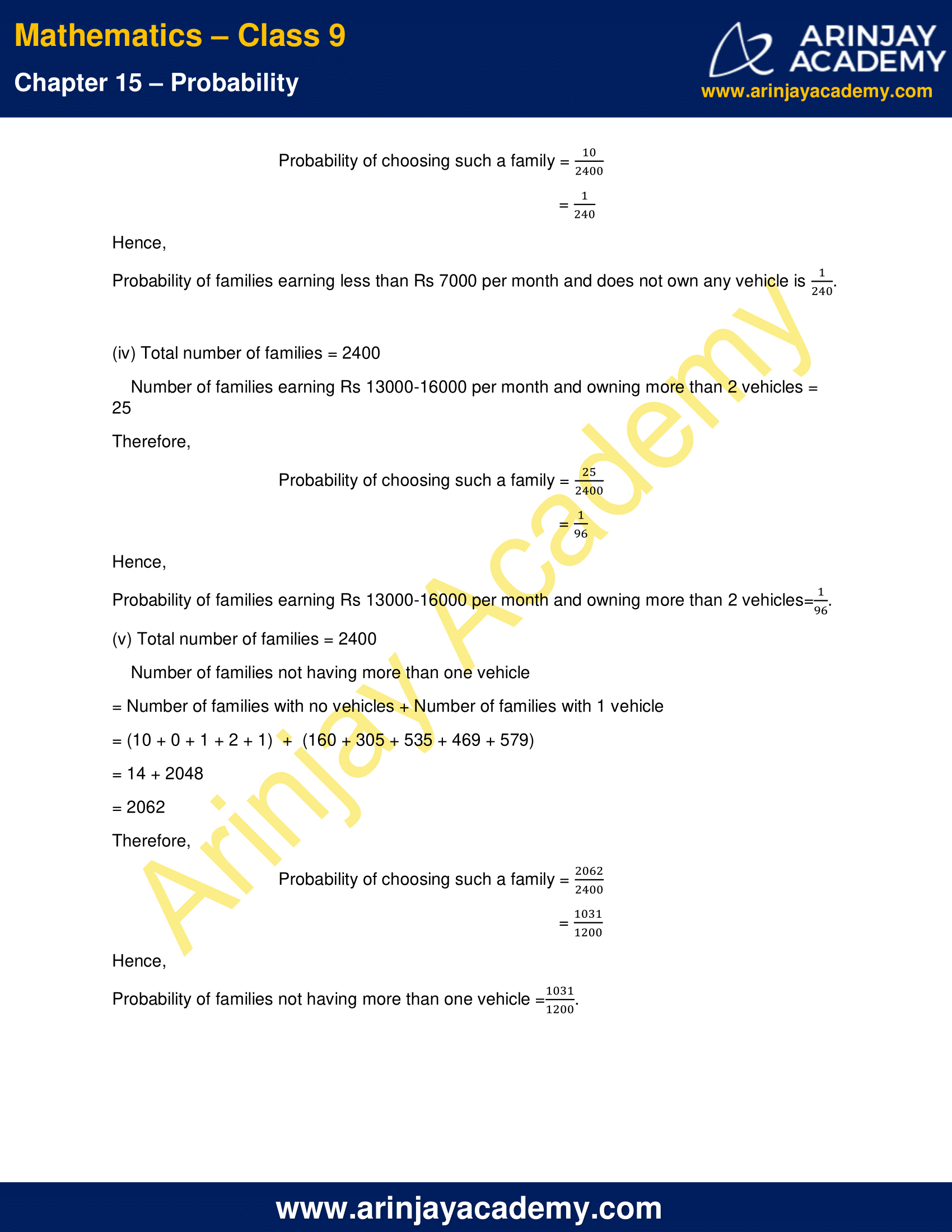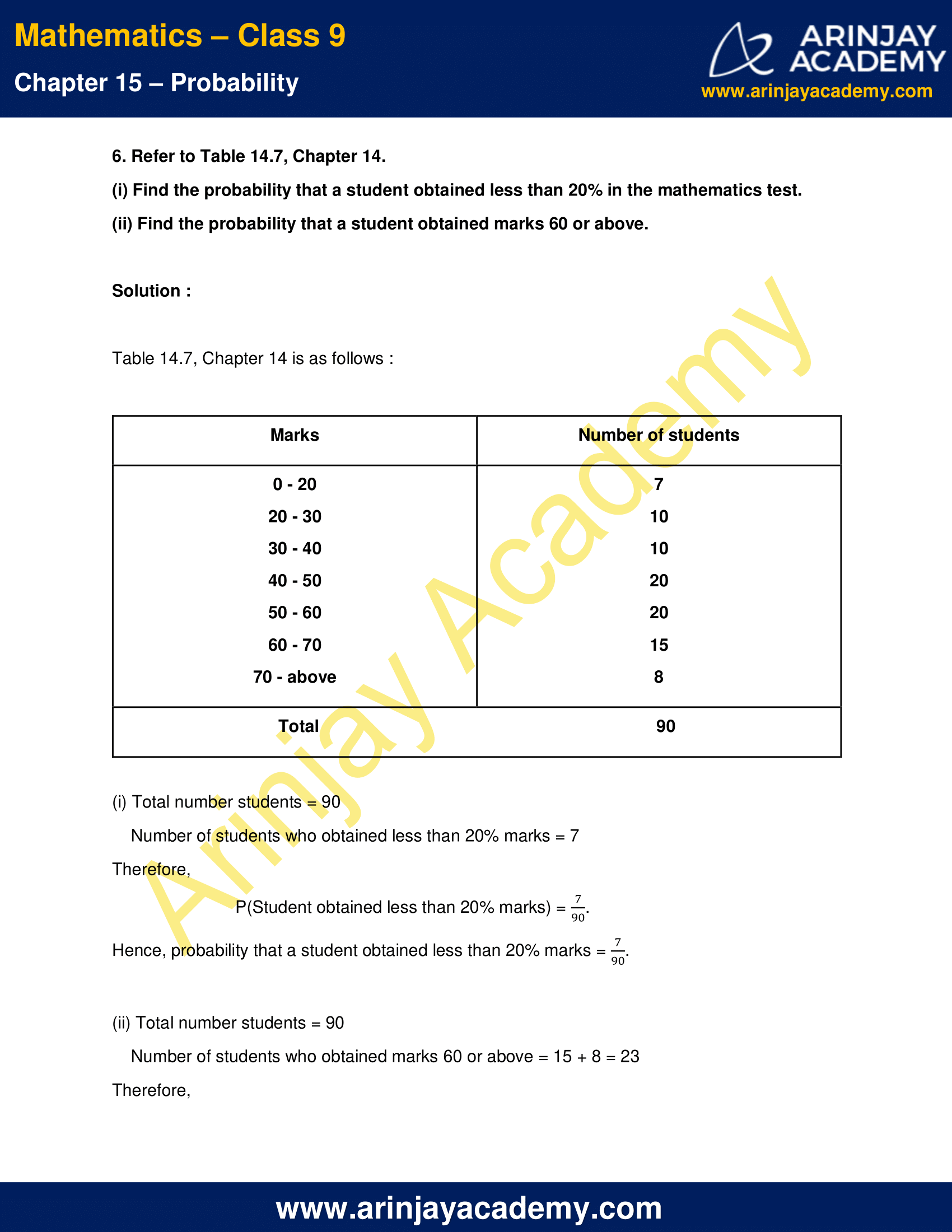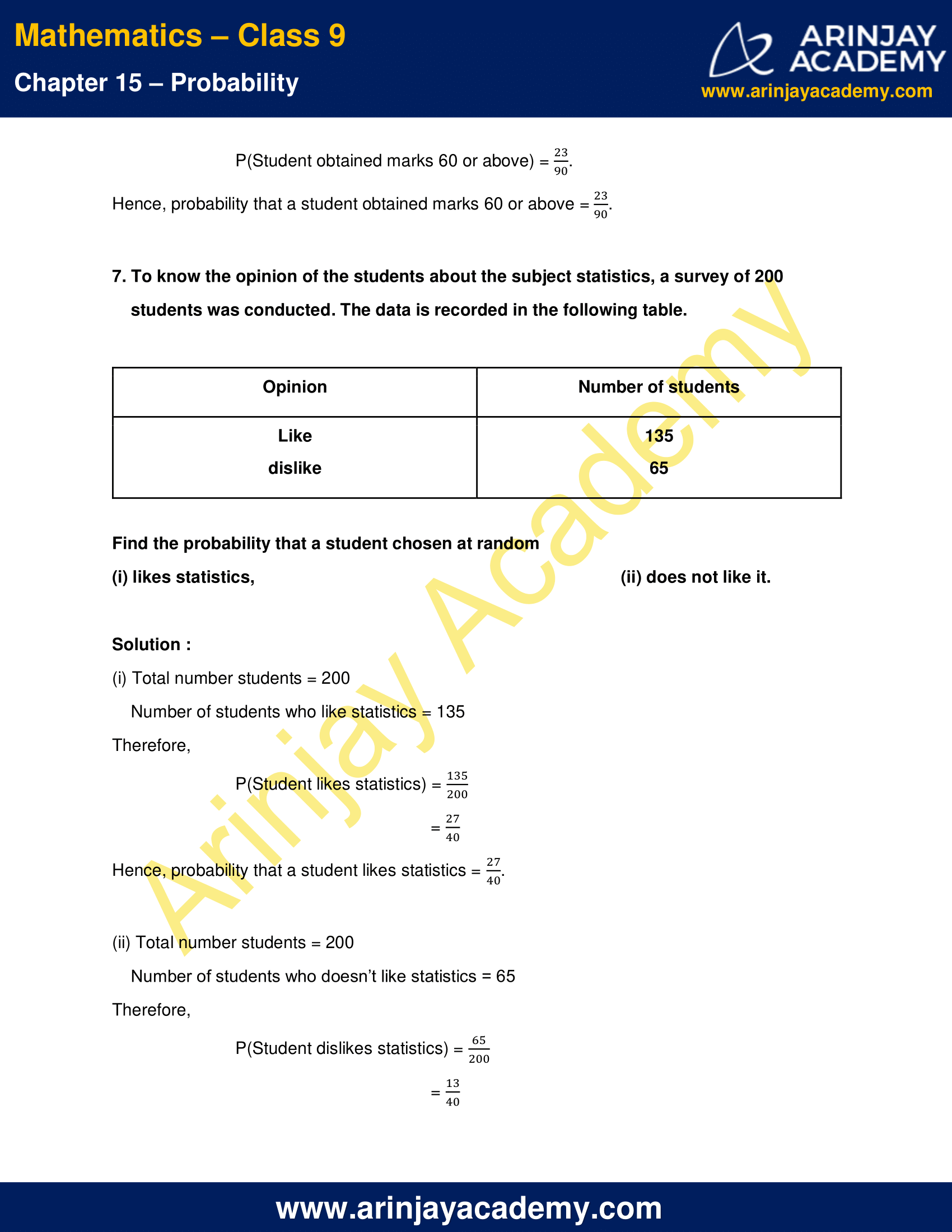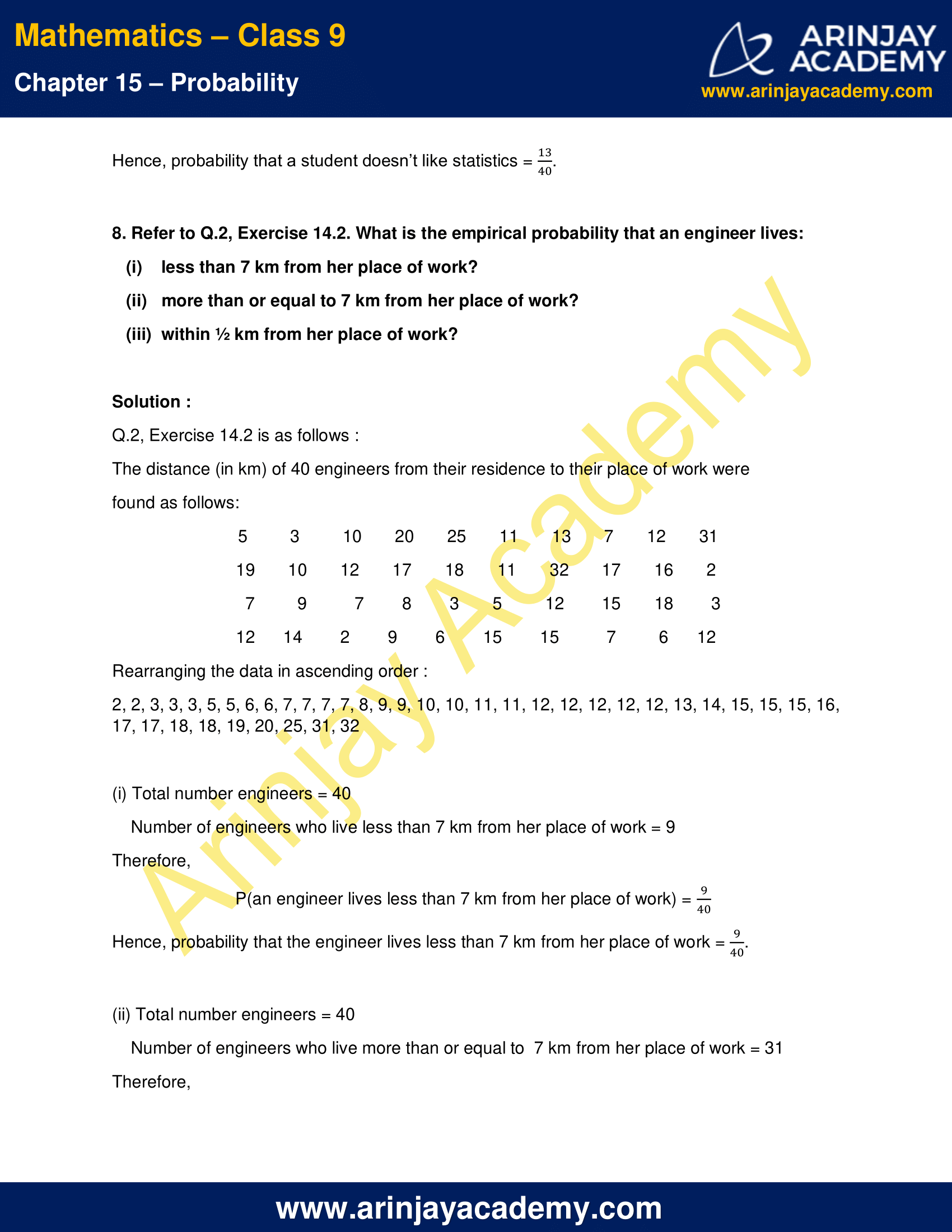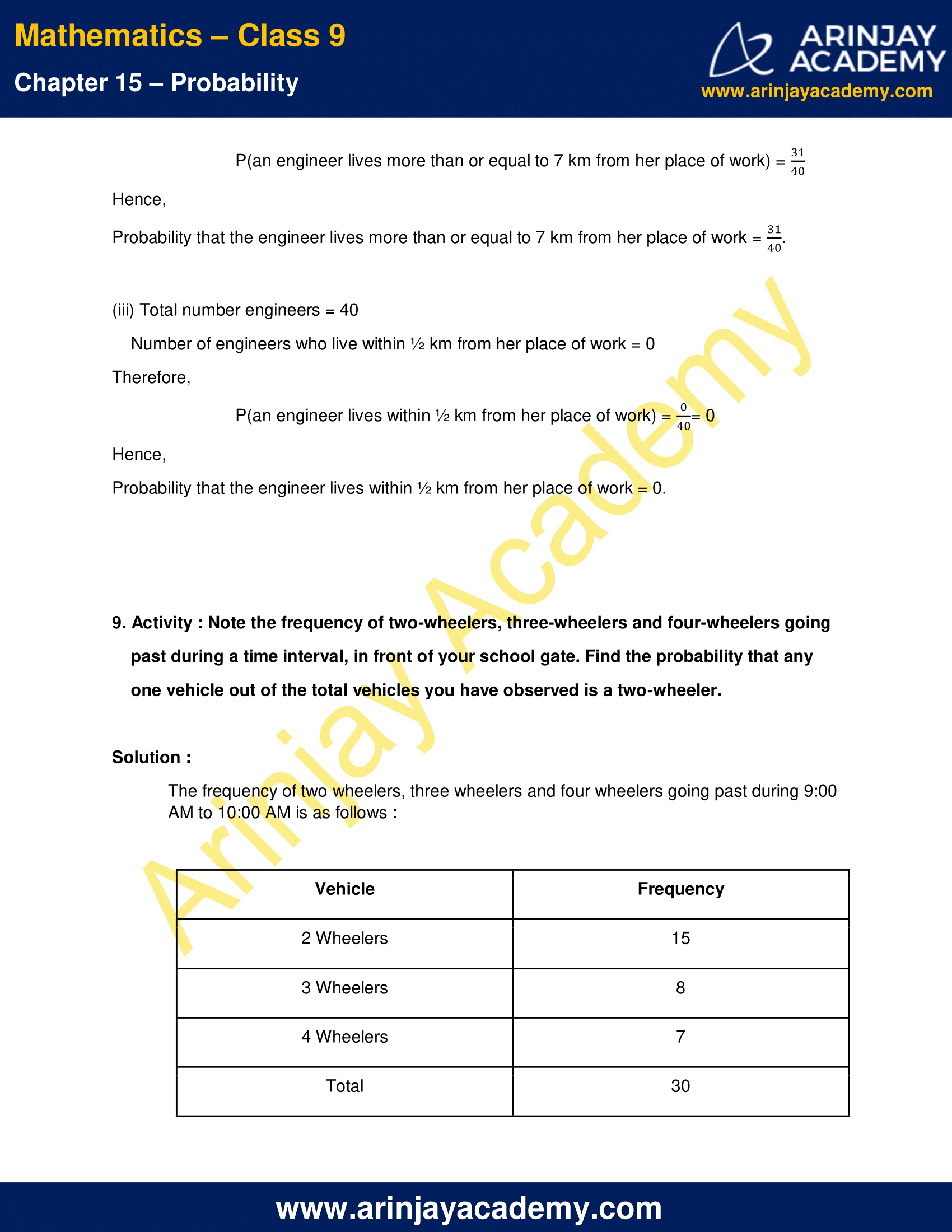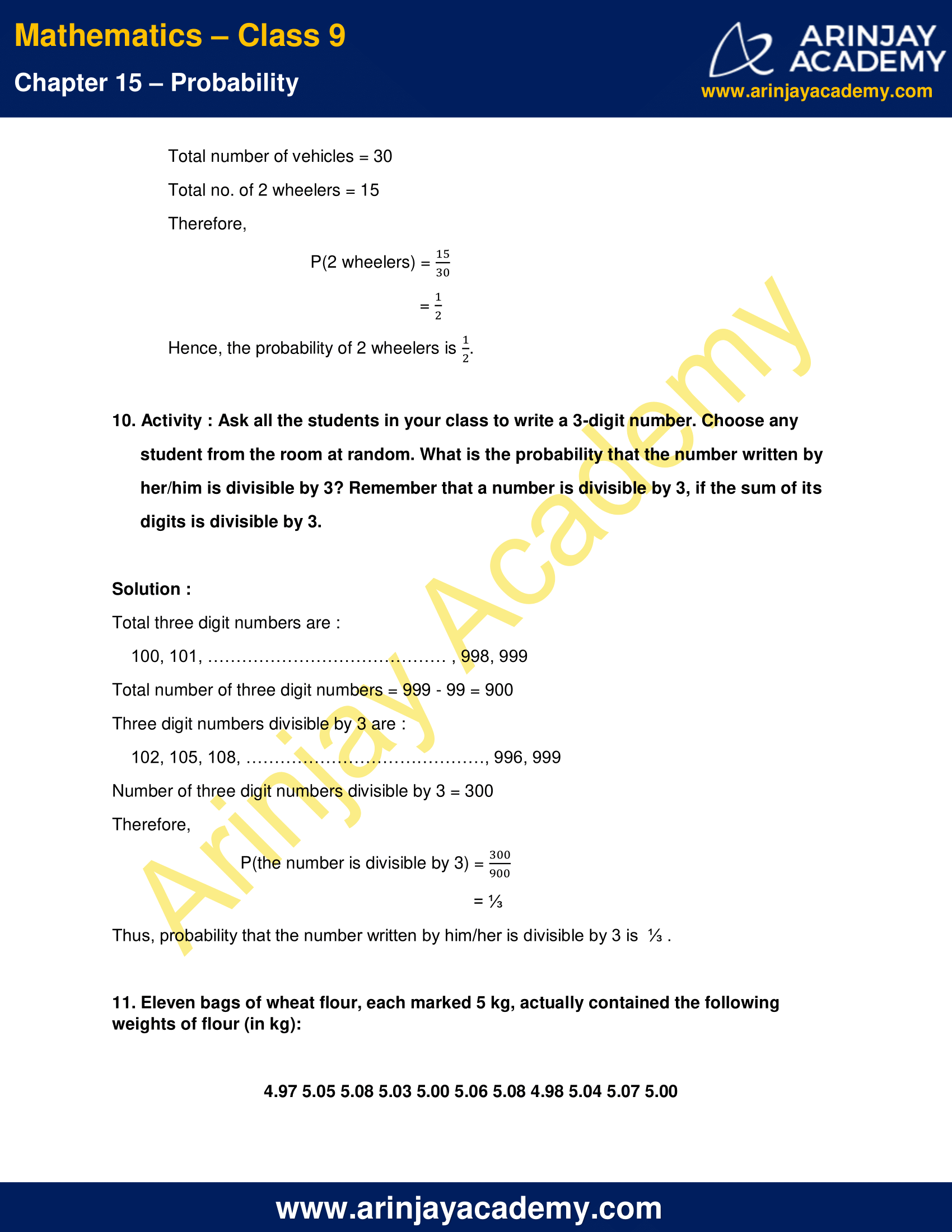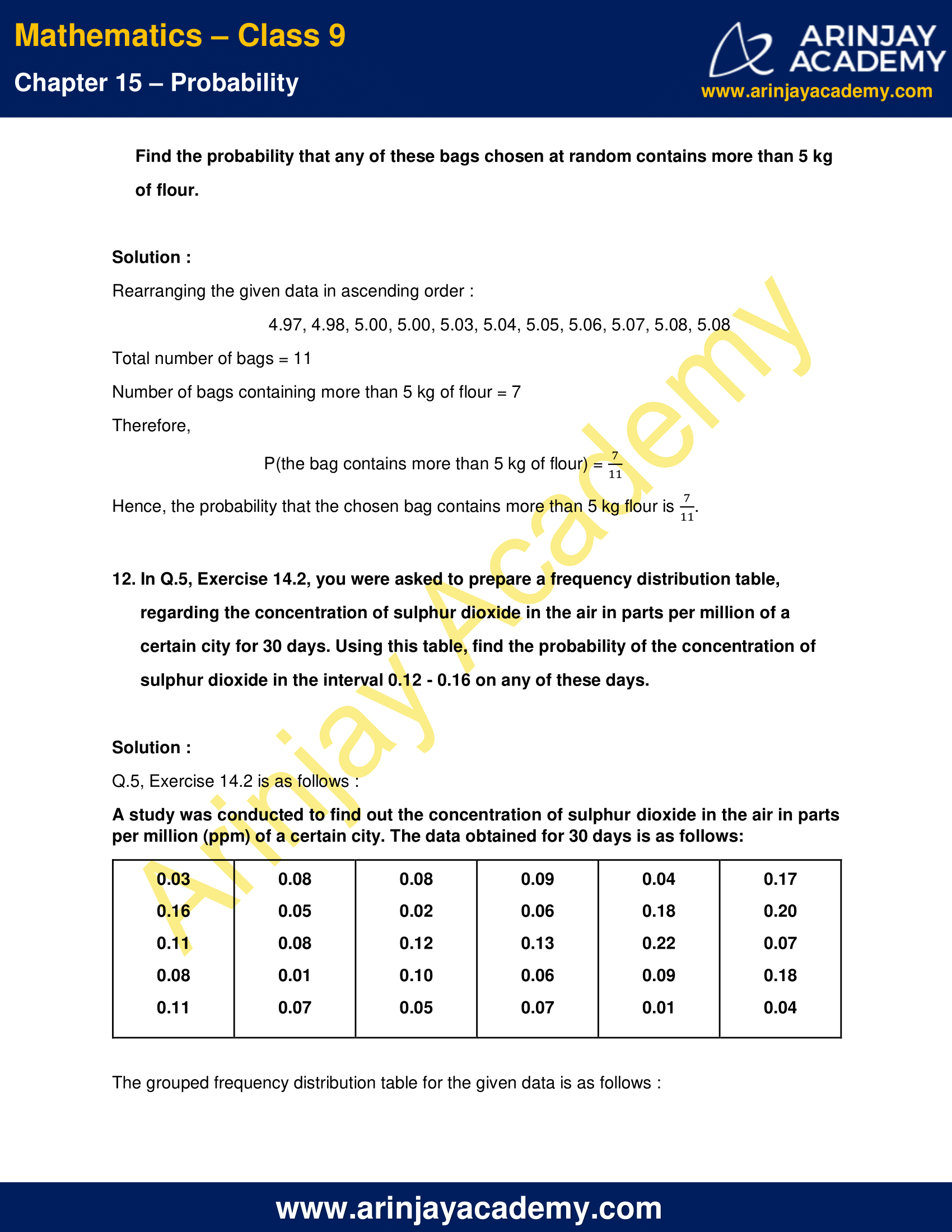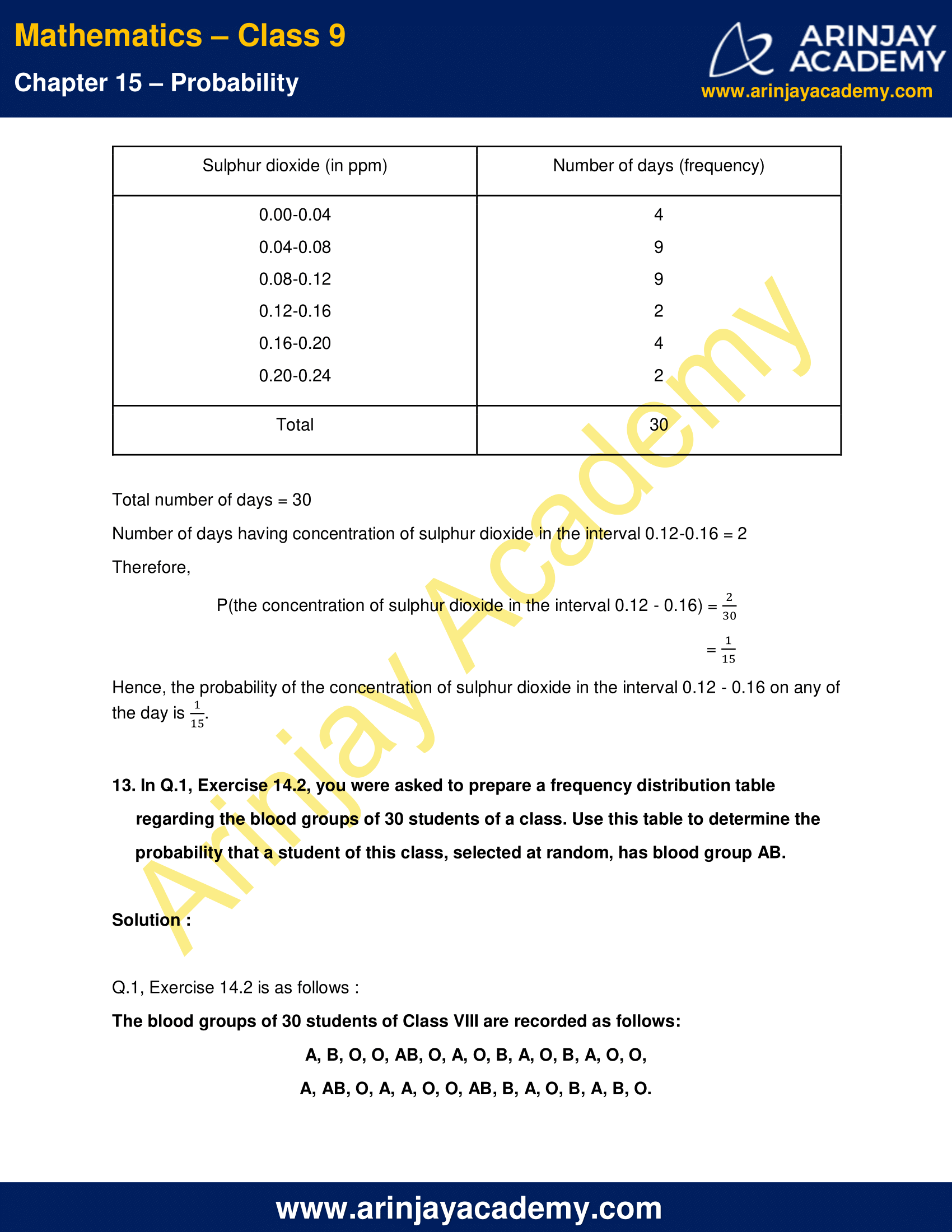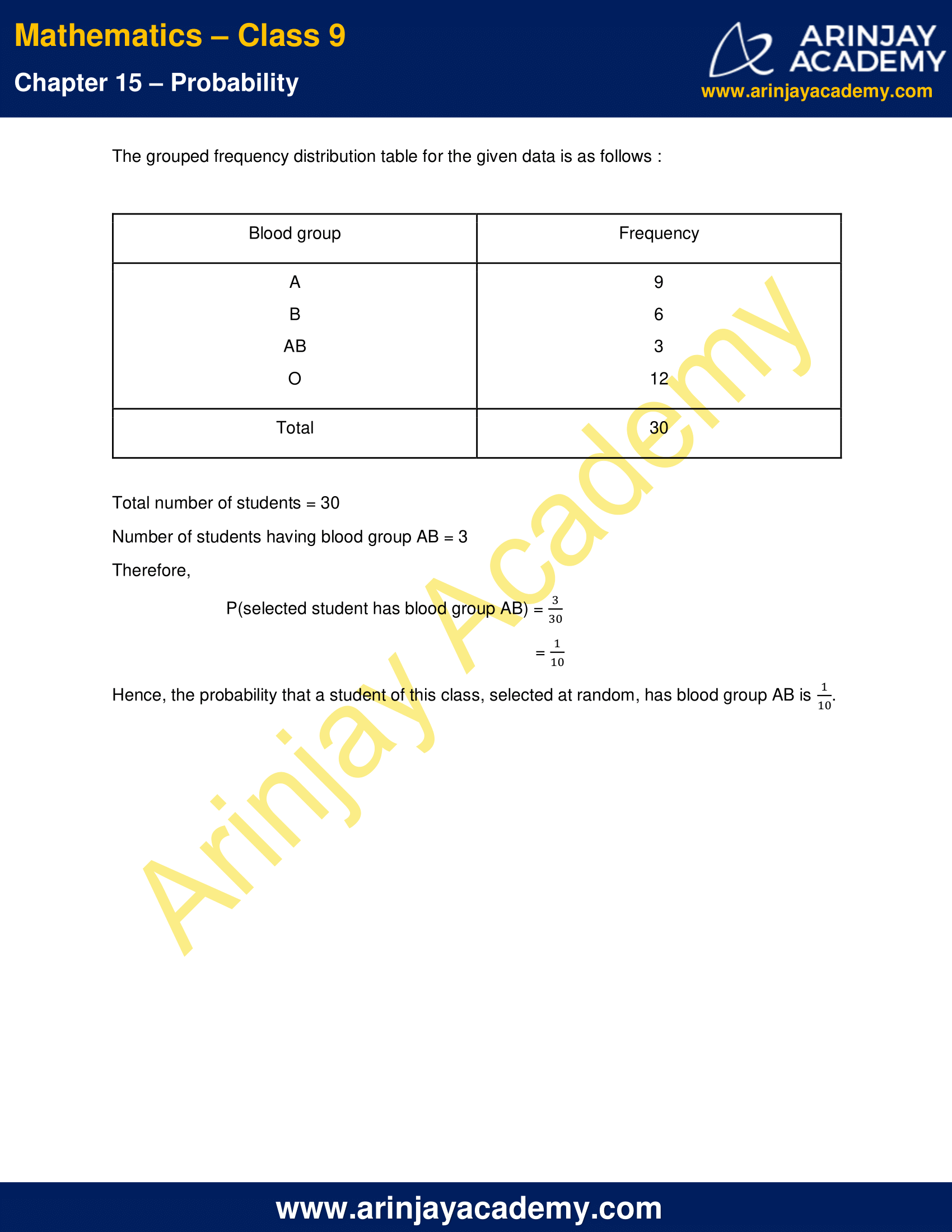NCERT Solutions for Class 9 Maths Chapter 15 Exercise 15.1 – Probability

1. In a cricket match, a batswoman hits a boundary 6 times out of 30 balls she plays. Find the probability that she did not hit a boundary.

Solution :

Total number of balls she played = 30
Number of balls she hits a boundary = 6
Therefore,
Number of balls she doesn’t hit a boundary
= 30 – 6
= 24
P(she did not hit a boundary) = 24/30
= 4/5
Hence, the probability of not hitting a boundary is 4/5.

2. 1500 families with 2 children were selected randomly, and the following data were recorded: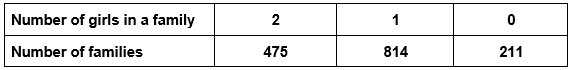Compute the probability of a family, chosen at random, having
(i) 2 girls
(ii) 1 girl
(iii) No girl
Also check whether the sum of these probabilities is 1.

Solution :

(i) Total number of families = 1500
Number of families having 2 girls = 475
P(2 girls) = 475/1500
= 19/60
Hence, the probability of a family having 2 girls is 19/60

(ii)Total number of families = 1500
Number of families having 1 girl child = 814
P(1 girls) = (814/1500)
= 407/750
Hence, the probability of a family having 1 girl is 407/750

(iii) Total number of families = 1500
Number of families having no girl = 211
P(no girls) = (211/1500)
Hence, the probability of a family having no girl is (211/1500).

Now, Sum of the probabilities obtained in parts (i), (ii) and (iii)
= (19/60) + (407/750) + (211/1500)
= (475+814+211)/1500
= (1500/1500)
= 1
Thus, we get the sum of their probabilities is 1.

3. Refer to Example 5, Section 14.4, Chapter 14(NCERT Class IX Mathematics). Find the probability that a student of the class was born in August.

Solution :

Given below is the Example 5, Section 14.4, Chapter 14(NCERT Class IX Mathematics):
In a particular section of Class IX, 40 students were asked about the months of their birth and the following graph was prepared for the data so obtained: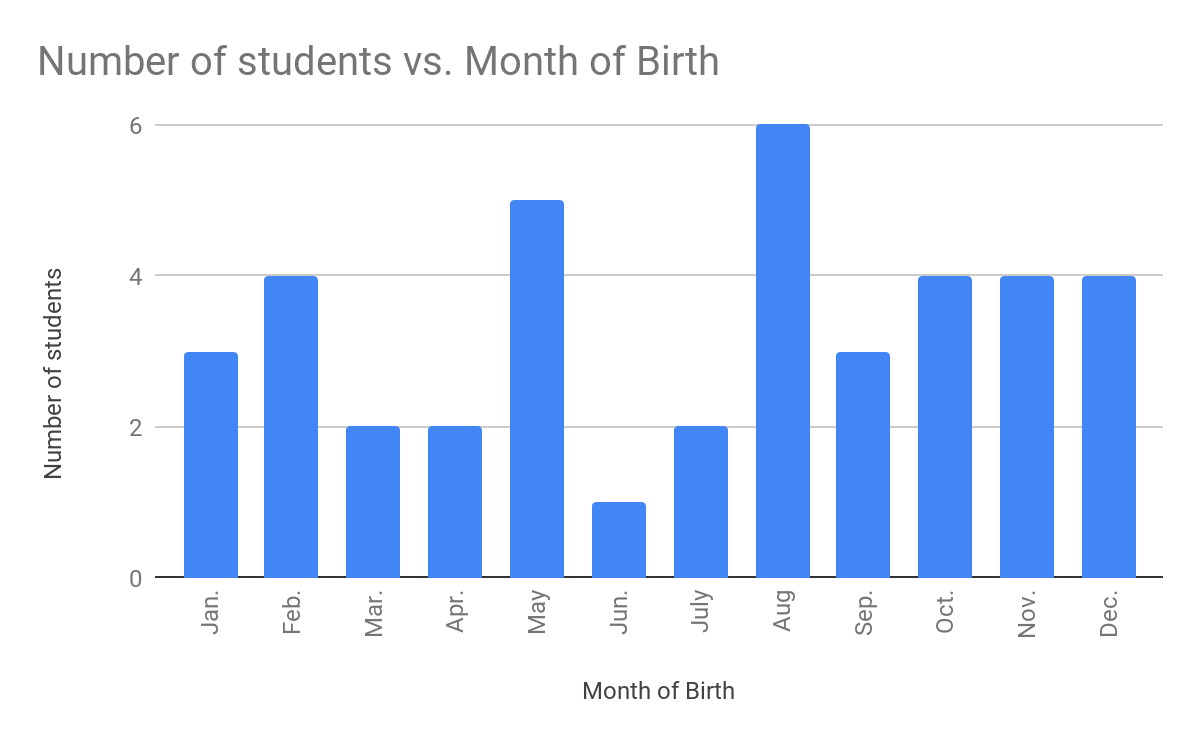Here,
Total number of students = 40
Number of students born in August = 6
P(Student was born in August) = 6/40
= 3/20
Hence, the probability that a student of the class was born in august is 3/20.

4. Three coins are tossed simultaneously 200 times with the following frequencies of different outcomes: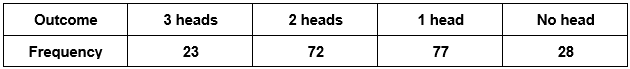If the three coins are simultaneously tossed again, compute the probability of 2 heads coming up.

Solution :

Number of total tosses = 200
Frequency of getting 2 heads = 72
P(2 heads) = 72/200
= 9/25
Hence, the probability of coming up 2 heads = 9/25

5. An organisation selected 2400 families at random and surveyed them to determine a relationship between income level and the number of vehicles in a family. The information gathered is listed in the table below: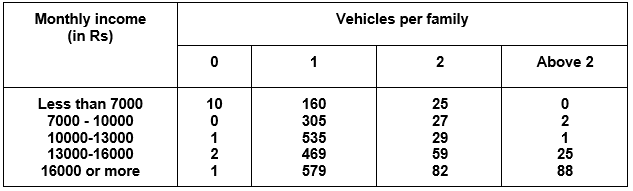Suppose a family is chosen. Find the probability that the family chosen is
(i)    earning Rs 10000 – 13000 per month and owning exactly 2 vehicles.
(ii)   earning Rs 16000 or more per month and owning exactly 1 vehicle.
(iii)  earning less than Rs 7000 per month and does not own any vehicle.
(iv)  earning Rs 13000 – 16000 per month and owning more than 2 vehicles.
(v)   owning not more than 1 vehicle.

Solution :

(i) Total number of families = 2400
Number of families earning Rs 10000-13000 per month and owning exactly 2 vehicles = 29
Probability of choosing such a family = 29/2400
Hence, Probability of families earning Rs 10000-13000 per month and owning exactly 2 vehicles is 29/2400.

(ii) Total number of families = 2400
Number of families earning Rs 16000 or more per month and owning exactly 1 vehicle = 579
Probability of choosing such a family = (579/2400)
Hence, Probability of families earning Rs 16000 or more per month and owning exactly 1 vehicle is (579/2400)

(iii) Total number of families = 2400

Number of families earning less than Rs 7000 per month and does not own any vehicle = 10
Probability of choosing such a family = (10/2400)
= (1/240)
Hence, Probability of families earning less than Rs 7000 per month and does not own any vehicle is 1/240.

(iv) Total number of families = 2400
Number of families earning Rs 13000-16000 per month and owning more than 2 vehicles = 25
Therefore, Probability of choosing such a family = (25/2400)
= (1/96)
Hence, Probability of families earning Rs 13000-16000 per month and owning more than 2 vehicles = 1/96

(v) Total number of families = 2400
Number of families not having more than one vehicle
= Number of families with no vehicles + Number of families with 1 vehicle
= (10 + 0 + 1 + 2 + 1)  +  (160 + 305 + 535 + 469 + 579)
= 14 + 2048
= 2062
Therefore, Probability of choosing such a family = (2062/2400)
= (1031/1200)
Hence, Probability of families not having more than one vehicle = (1031/1200)

6. Refer to Table 14.7, Chapter 14.

(i) Find the probability that a student obtained less than 20% in the mathematics test.
(ii) Find the probability that a student obtained marks 60 or above.

Solution :

Table 14.7, Chapter 14 is as follows :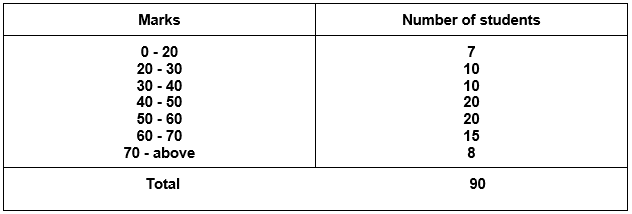(i) Total number students = 90
Number of students who obtained less than 20% marks = 7
P(Student obtained less than 20% marks) = 7/90
Hence, probability that a student obtained less than 20% marks = 7/90

(ii) Total number students = 90
Number of students who obtained marks 60 or above = 15 + 8 = 23
P(Student obtained marks 60 or above) = 23/90
Hence, probability that a student obtained marks 60 or above = 23/90

7. To know the opinion of the students about the subject statistics, a survey of 200 students was conducted. The data is recorded in the following table.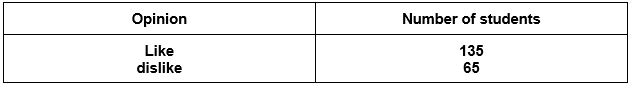Find the probability that a student chosen at random

(i) likes statistics,
(ii) does not like it.

Solution :

(i) Total number students = 200
Number of students who like statistics = 135
P(Student likes statistics) = 135/200
= 27/40
Hence, probability that a student likes statistics = 27/40

(ii) Total number students = 200
Number of students who doesn’t like statistics = 65
P(Student dislikes statistics) = 65/200
= 13/40
Hence, probability that a student doesn’t like statistics = 13/40

8. Refer to Q.2, Exercise 14.2. What is the empirical probability that an engineer lives:
(i)    less than 7 km from her place of work?
(ii)   more than or equal to 7 km from her place of work?
(iii)  within ½ km from her place of work?

Solution :

Q.2, Exercise 14.2 is as follows :
The distance (in km) of 40 engineers from their residence to their place of work were found as follows: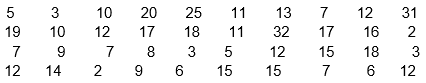Rearranging the data in ascending order :
2, 2, 3, 3, 3, 5, 5, 6, 6, 7, 7, 7, 7, 8, 9, 9, 10, 10, 11, 11, 12, 12, 12, 12, 12, 13, 14, 15, 15, 15, 16, 17, 17, 18, 18, 19, 20, 25, 31, 32

(i) Total number engineers = 40
Number of engineers who live less than 7 km from her place of work = 9
P(an engineer lives less than 7 km from her place of work) = 9/40
Hence, probability that the engineer lives less than 7 km from her place of work = 9/40

(ii) Total number engineers = 40
Number of engineers who live more than or equal to  7 km from her place of work = 31
P(an engineer lives more than or equal to 7 km from her place of work) = 31/40
Hence, Probability that the engineer lives more than or equal to 7 km from her place of work = 31/40

(iii) Total number engineers = 40
Number of engineers who live within ½ km from her place of work = 0
P(an engineer lives within ½ km from her place of work) = 0/40 = 0
Hence, Probability that the engineer lives within ½ km from her place of work = 0.

9. Activity : Note the frequency of two-wheelers, three-wheelers and four-wheelers going past during a time interval, in front of your school gate. Find the probability that any one vehicle out of the total vehicles you have observed is a two-wheeler.

Solution :

The frequency of two wheelers, three wheelers and four wheelers going past during 9:00 AM to 10:00 AM is as follows :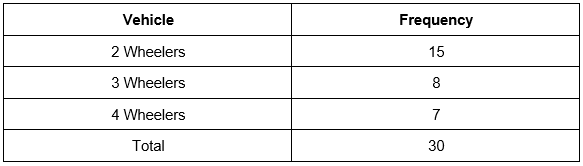Total number of vehicles = 30
Total no. of 2 wheelers = 15
P(2 wheelers) = 15/30
= 1/2
Hence, the probability of 2 wheelers is 1/2

10. Activity : Ask all the students in your class to write a 3-digit number. Choose any student from the room at random. What is the probability that the number written by her/him is divisible by 3? Remember that a number is divisible by 3, if the sum of its digits is divisible by 3.

Solution :

Total three digit numbers are :
100, 101, …………………, 998, 999
Total number of three digit numbers = 999 – 99 = 900
Three digit numbers divisible by 3 are :
102, 105, 108, …………………, 996, 999
Number of three digit numbers divisible by 3 = 300
P(the number is divisible by 3) = 300/900
= 1/3
Thus, probability that the number written by him/her is divisible by 3 is 1/3

11. Eleven bags of wheat flour, each marked 5 kg, actually contained the following weights of flour (in kg):

4.97, 5.05, 5.08, 5.03, 5.00, 5.06, 5.08, 4.98, 5.04, 5.07, 5.00

Find the probability that any of these bags chosen at random contains more than 5 kg of flour.

Solution :

Rearranging the given data in ascending order :
4.97, 4.98, 5.00, 5.00, 5.03, 5.04, 5.05, 5.06, 5.07, 5.08, 5.08
Total number of bags = 11
Number of bags containing more than 5 kg of flour = 7
P(the bag contains more than 5 kg of flour) = 7/11
Hence, the probability that the chosen bag contains more than 5 kg flour is 7/11

12. In Q.5, Exercise 14.2, you were asked to prepare a frequency distribution table, regarding the concentration of sulphur dioxide in the air in parts per million of a certain city for 30 days. Using this table, find the probability of the concentration of sulphur dioxide in the interval 0.12 – 0.16 on any of these days.

Solution :

Q.5, Exercise 14.2 is as follows :
A study was conducted to find out the concentration of sulphur dioxide in the air in parts per million (ppm) of a certain city. The data obtained for 30 days is as follows: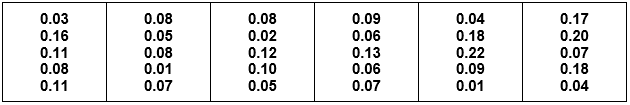The grouped frequency distribution table for the given data is as follows :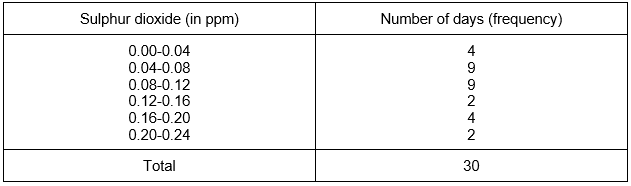Total number of days = 30
Number of days having concentration of sulphur dioxide in the interval 0.12-0.16 = 2
P(the concentration of sulphur dioxide in the interval 0.12 – 0.16) = 2/30
= 1/15
Hence, the probability of the concentration of sulphur dioxide in the interval 0.12 – 0.16 on any of the day is 1/15

13. In Q.1, Exercise 14.2, you were asked to prepare a frequency distribution table regarding the blood groups of 30 students of a class. Use this table to determine the probability that a student of this class, selected at random, has blood group AB.

Solution :

Q.1, Exercise 14.2 is as follows :
The blood groups of 30 students of Class VIII are recorded as follows:
A, B, O, O, AB, O, A, O, B, A, O, B, A, O, O, A, AB, O, A, A, O, O, AB, B, A, O, B, A, B, O.
The grouped frequency distribution table for the given data is as follows :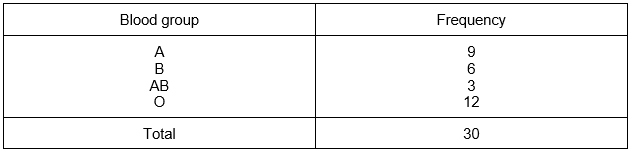Total number of students = 30
Number of students having blood group AB = 3
P(selected student has blood group AB) = 3/30
= 1/10
Hence, the probability that a student of this class, selected at random, has blood group AB is 1/10

NCERT Solutions for Class 9 Maths Chapter 15 Exercise 15.1 – Probability, has been designed by the NCERT to test the knowledge of the student on the topic – 2 Probability – an Experimental Approach

Download NCERT Solutions for Class 9 Maths Chapter 15 Exercise 15.1 – Probability